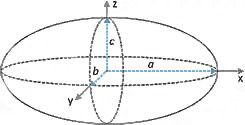# Ellipsoid Volume Calculator

This Ellipsoid Volume Calculator is designed to help you calculate the volume of an ellipsoid.

To use the calculator, simply enter all the required values and click "Calculate". A running total will also be displayed as you enter new dimensions into the volume calculator.## General information and basic definition of an ellipsoid

An ellipsoid represents a three-dimensional solid analogue of a geometric figure known as an ellipse, and its surface can be described as quadratic. In Cartesian coordinates, the general equation describing the quadratic surface of an ellipsoid is as follows:

(x / a)2 + (y / b)2 + (z / c)2 = 1

The following formula can be applied to calculate the volume of an ellipsoid:

• Volume (V) = (4/3) × π × a × b × c
• where:
• a, b, and c are the lengths of the semi-axes;
• π is 3.141592654 (to 9 decimal places).

Note: Note: Units are provided for convenience purposes only and do not affect the calculations.

Units used to measure the volumes of solid objects are:

• cubic feet
• cubic meters
• cubic yards

Units used to measure the volumes of liquids are:

• liters
• quarts
• pints
• gallons